# Multiplication + addition - math problems

#### Number of problems found: 121

• Product and sumWhat is the product of two fourths  and the sum of three halves and four?
• The libraryThere are 65 boxes of books. Each box contains 100 books. If each book costs 19.75 how much is the total cost of all the books?
• Nice two numbersWhat two numbers we give a sum of 30 difference of 24 products of 81 and a quotient of 9?
• The space museum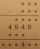The science club is going to the space museum. The club consists of 27 students. If admission costs \$6.50 for children ages 4–11 and \$8.50 for children ages 12–17, how much will it cost for the entire science club's admission to the museum? Science Club S
• Nathan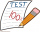Nathan is playing a trivia game. Each correct answer is worth 2 ½ points, and each incorrect answer is worth -¼ points. If he gets 35 questions correct and 32 questions incorrect, how many points does he have? A. 87.5 B. 79.5 C. 75 D. 8
• 30 eggs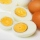There are 30 eggs in a tray. If 1/2 of the tray used 1/5 of it cooked,1/3 kept the refrigerator, how many eggs were left?
• Translate 2Translate the given phrases to mathematical phrases. Thrice the sum of three fifths and two thirds less one half is what number?
• A land 2A land owner owns two parcels of land measuring 4 2/5 hectares and 6 1/5 hectares. He sells 3/4 of the total land. How many hectares of the land are left?
• Simplify expression with mixedWhen (7¹/3 + 2¾)÷(2 + 2¼ × 1¹/3) is simplified the result is?
• Evaluate 17Evaluate 2x+6y when x=- 4/5 and y=1/3. Write your answer as a fraction or mixed number in simplest form.
• Evaluate fractionsThe difference  of 5 1/2 and 2/3 is added to the product of 5/6 and 1/2
• Sign of a expressionWhat is the sign of expression: minus 18 start fraction 9 divided by 17 end fraction plus left parenthesis minus 18 start fraction 9 divided by 17 end fraction right parenthesis?
• Which 4Which number is equivalent to 5×10+4×1+8×110+6×1100+1×11,000?
• Product increased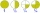When the product of 2/3 and 6/10 is increased by 2/5, the result is?
• Quotient and productIf the quotient of [8/5 divided by 8/10] is added to the product of [8/14 x 7/12 x 3/8], what is the sum?
• Simplify 7Simplify. 7-1/3÷ 3-2/3 of 2+ 4-1/2÷ 2-1/4+ 1/2 solution and by step by step
• The cost 2The cost of 5 apples is \$3.45  and 5 oranges is \$1.23. If Rachel buys one apple and one orange, then how much must she pay?
• Complicated sum minus productWhat must be subtracted from the sum of 3/8 and 5/16 to get difference equal to the product of 5/8 and 3/16?
• Groceries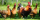Arturo buys 2 cans of tomato soup that cost \$0.52 each and 12 cans of chicken noodle soup that cost \$0.51 each. How much is the total cost of these groceries?
• Flower gardenIn the mr elliots garden 1/8 of the flowers are red 1/4 of them are purple and 1/4 of the remaning flowers are pink. If there is 128 flowers how many of them are pink?

Do you have an exciting math question or word problem that you can't solve? Ask a question or post a math problem, and we can try to solve it.

We will send a solution to your e-mail address. Solved examples are also published here. Please enter the e-mail correctly and check whether you don't have a full mailbox.

Please do not submit problems from current active competitions such as Mathematical Olympiad, correspondence seminars etc...